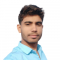## Plotting Zeros (Max Term Representation) of a Boolean Function on K-MapUpdated on 03-Oct-2023 13:53:32

35 Views

The K-Map or Karnaugh Map is a systematic method of simplifying a complex Boolean function or expression. The K-Map is basically a graph or a chart which consists of a certain number of adjacent cells. Each cell represents a particular combination of variables in either sum or product form. However, we can use the K-Map for simplifying a Boolean function in any number of variables, but it becomes tedious for functions involving five or more variables. In actual practice, we usually use K-Map for simplification of Boolean functions in upto six variables. A Boolean function in n variables can have ... Read More

## Don't Care (X) Conditions in K-MapsUpdated on 03-Oct-2023 12:38:38

554 Views

K-Map or Karnaugh Map is a graphical method of simplifying Boolean expression. A K-Map composed of an arrangement of adjacent squares or cells, where each cell represent a particular combination of variables in sum or product form. In the K-map method, there is a useful condition namely, Don’t Care Condition, which helps in simplifying a Boolean function. The don’t care condition makes the grouping of variables in K-map easy. In this tutorial, we will understand the "don’t care" concept in K-map reduction with the help of solved examples. Sometimes, in a Boolean expression for certain input combinations, the value of ... Read More

## 5 Variable K-Map in Digital ElectronicsUpdated on 03-Oct-2023 12:01:23

597 Views

K-Map or Karnaugh Map is a simplification technique used to minimize a given complex Boolean function. K-Map or Karnaugh Map is a graph or chart which is composed of an arrangement of adjacent cells, where each cell of the K-Map represents a particular combination of variables in either sum or product form. The K-map can be used to simplify Boolean functions involving any number of variables. But, the simplification of a Boolean function using K-map becomes very complex for expressions involving five or more variables. Therefore, in actual practice, the K-map is limited to six variables. The number of cells ... Read More

## 4 Variable K-Map in Digital ElectronicsUpdated on 05-Sep-2023 16:58:46

2K+ Views

Several techniques have been developed to simplify a complex Boolean expression into its simplest form. K-Map or Karnaugh Map is one of such minimization or simplification techniques. The K-Map or Karnaugh Map is a graph or chart which composed of an arrangement of adjacent cells. Where, each cell of the K-Map represents a particular combination of variables in either sum or product form. The K-map can be used to simplify Boolean functions involving any number of variables. But, the simplification of a Boolean function using K-map becomes tedious for problems involving five or more variables. Therefore, in actual practice, the ... Read More

## Simplifying a Boolean Expression using K-MapUpdated on 19-Apr-2023 15:48:25

6K+ Views

Karnaugh Map (K-Map) K-Map is a graphical tool used for simplifying Boolean expressions represented in their standard form to obtain their minimal form. The K-Map is basically a graph or chart that composed of an arrangement of adjacent cells or squares, where each cell represents a particular combination of variables of the function either in sum or product form. The number of cells in the K-Map depends upon number of variables in the Boolean function, i.e., K-map has 2n adjacent cells, where n is the number of variables in the Boolean expression. Therefore, the number of cells in a 2 ... Read More

## 6 Variable K-Map in Digital ElectronicsUpdated on 19-Apr-2023 10:45:01

2K+ Views

Read this article to learn how you can use the K-Map (Karnaugh Map) to reduce a Boolean function in six variables. Let's start with a brief introduction to Karnaugh Map (K-Map). Karnaugh Map (K-Map) The Karnaugh Map or K-Map is a graphical method of reducing a Boolean function to its minimal form. The K-Map can be defined as a chart or a graph that is composed of an arrangement of adjacent squares or cells, where each cell represents a particular combination of variables of the Boolean expression either in sum or product form. A typical 2 Variable K-Map is represented ... Read More

## 3 Variable K-Map in Digital ElectronicsUpdated on 26-Dec-2022 17:31:17

4K+ Views

A K-Map or Karnaugh Map is a graphical method that used for simplifying the complex algebraic expressions in Boolean functions. This method avoid the use of complex theorems and equations manipulations. A K-Map is basically a special form of a truth table that can easily map out the values of parameters and gives a simplified Boolean expression. K-Map method is best suited for such Boolean functions that have two to four variables. However, it can be used for Boolean functions having five or six variables as well, but its process becomes more difficult with the increased number of variables in ... Read More